Learning Library

Speedy Rounding and Subtracting

With the help of these cute critters, your kid will be rounding off and subtracting numbers like a pro!Rounding Numbers: Subtraction

As your second grader learns to round up or down and then complete subtraction equations, his overall maths skills will improve.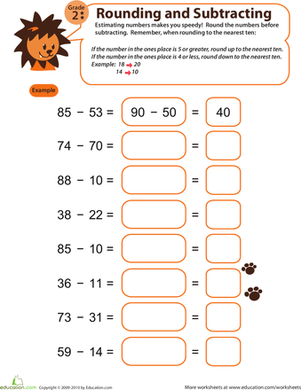Speedy Rounding and Subtracting 2

Watch your year three's estimation and subtraction skills skyrocket with this fun rounding and subtracting worksheet!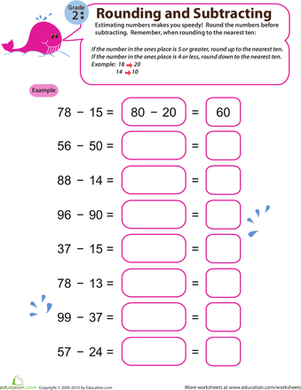Speedy Rounding and Subtracting 3

With sufficient estimation practise, your second grader will gain a better understanding of numbers and significantly improve her subtraction skills!Speedy Rounding and Subtracting 4

Sharpen your second grader's ability to subtract quickly and efficiently by integrating estimation into the process with this adorable worksheet.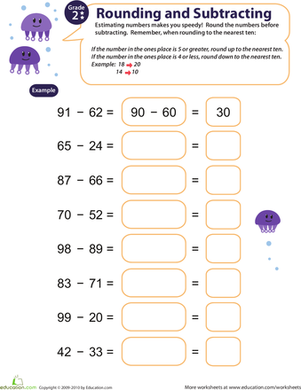Speedy Rounding and Subtracting 5

With this adorable worksheet, your second grader will gain a better understanding of numbers and learn how to estimate subtraction problems quickly.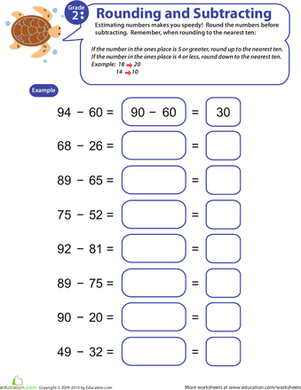Speedy Rounding and Subtracting 6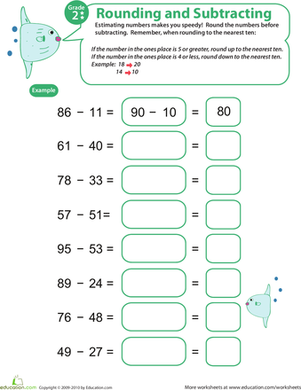Speedy Rounding and Subtracting 7

Introduce your second grader to this cute worksheet, and watch her estimation and subtraction skills skyrocket!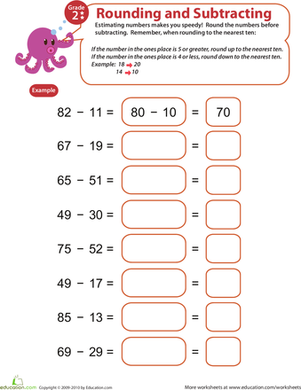Speedy Rounding and Subtracting 8

We're willing to bet that your second grader will learn how to subtract quickly and efficiently by incorporating estimation into the process!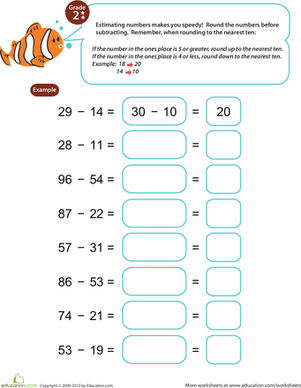Speedy Rounding and Subtracting 9

As your second grader practices working with rounded numbers, he will improve not only his subtraction, but his overall maths skills as well.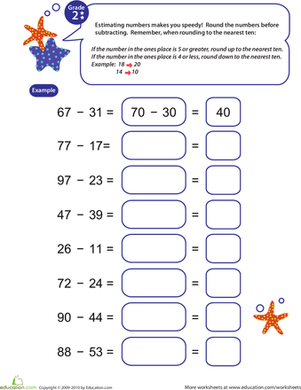Speedy Rounding and Subtracting 10

Improve your second grader's subtraction and estimation skills with this charming, colorful worksheet.

Create new collection

0

New Collection>

0Items

What could we do to improve Education.com?